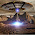## Oct 22, 2013

### Discrete Mathematics Problem - Grouping Students

Source: Sent to me by Vinayak Gagrani (CSE IITB Alumnus 2013)

Problem:

There are 289 students. We have to divide them into 17 groups of 17 each every day. The groups have to be such that no two students who have been previously on some group together can be formed a group again. How many days can we do this ?

Extension:
Can this be generalized for any N^2 students?

1.18 days. Each person sees 16 different people on each day, so 288 different people in 18 days. In the 19th day he will see more than 288, so it's not possible.
Groups are numbered 0,1,2,...16. People are numbered 0,1,...288.
On day 0, person i is in group floor(i/17).
On day j (for j=1,2,...17), group k contains persons 17m+ [k+(m(j-1))%17] for m=0,1,2,...16.The % operator is modulo division.
We need to show: 1) On each day, each group has 17 distinct people and each pair of groups is disjoint. 2) Each person is grouped with every other person exactly once.
To show 1): Suppose 17m+ [k+(m(j-1))%17] = 17m'+[k'+(m'(j-1))%17]
Then m'=m and k'=k. This shows that each person is distinct on a particular day.
To show 2): Suppose that the m1_th person and m2_th person in group k of day j also occur together in group k' of day j' as the m1'-th and m2'-th persons respectively. Then
17m1+ [k+(m1(j-1))%17] = 17m1'+[k'+(m1'(j'-1))%17] and
17m2+ [k+(m2(j-1))%17] = 17m1'+[k'+(m2'(j'-1))%17]
Therefore, m1=m1',
k+m1(j-1) = k'+m1(j'-1) (mod 17) and
k+m2(j-1) = k'+m2(j'-1) (mod 17)
Subtracting the last 2 congruences gives
(m1-m2)(j-1)=(m1-m2)(j'-1) (mod 17)
thus 17 divides (m1-m2)(j-j')
but 17 is prime, and |m1-m2|, |j-j'| are both between 1 and 16. Hence, it's a contradiction.

The approach works for n^2 people when n is prime. I am not sure if it can be generalized for composite n.

2.N+1;

Note that, A guy finishes his grouping stint with N-1 students in each day and there are N^2-1 students other than the guy himself. So there are maximum (N^2-1)/(N-1) = N+1 days are possible before he exhaust grouping with all the students. Now, you can show N+1 is achievable as follows :

First day
1,2,3
4,5,6
7,8,9
2nd day (transpose)
1,4,7
2,5,8
3,6,9
Now in 3rd day, you group members diagonally from previous one ( cyclically)
(for example if you notice main diagonal is [1,5,9] , you take it, similarly for [2,6 and wrapped around to 7]
1,5,9
2,6,7
3,4,8

Group elements diagonally again
1,6,8
2,4,9
3,5,7

So there will be initial matrix, its transpose with N possible diagonal rotation, hence total N+1 days possible.

3.18 days...
For N^2 students, number of days we can do this is (N+1).

4.Only N+1 days as if there are four students ABCB, then only groups possible is
{AB,CD}, {AC,BD}, {AD,BC} i.e. 2+1=3 days. if there are 9 students 1,2,3,4,5,6,7,8,9
then possible configuration will be
[123,456,789],
[147,258,369],
[159,357,168],
[249,267,384]
i.e.3+1=4 days and so on,
so for N^2 students making N group each day having N students in each group without having 2 students in same group gain we can adjust only for N+1 days

5.For general N, this the number of days you can do this is equal to 2 plus the maximum number of mutually orthogonal Latin Squares of order N. When N is any prime power, this means we can do this for N+1 days, but for most N, this is a hard open problem.

6.This comment has been removed by the author.

7.N+1-(no. of factors of N except 1 and N)

1.For 6*6 students, how do you do this for 5 days?

8.The answer is (n+1).
Example: take n = 3. And you form three groups for 9 students. Let they are numbered from 1, 2,..., 9.
The first group. Let call it Grouping A.
1 2 3 | 4 5 6 | 7 8 9
Now you can just use the positions of the students. Suppose you put a difference of 0 positions. That means you take first student from each of the Grouping A to form the first group. Then you take second student from each group for the second group. And so for the third. This will give:
1 4 7 | 2 5 8 | 3 6 9
Now, you take the difference of 1 position. Now, to create the first group you take first student from first group of Grouping A, then second student from the second group of grouping A, then the third student from the third group of Grouping A. This will give:
1 5 9 | 2 6 7 | 3 4 8
Now, you put a difference of 2 positions. This will give
1 6 8 | 2 4 9 | 3 5 7
In this way you have 4 groupings.

So, for generalized case you can put difference of 0 ,1 , 2.... till (n-1) after you form the Grouping A like done in the example.
So, the answer would be (n+1).

### Fraction Brainteaser

Source: Sent to me by Gaurav Sinha Problem: Siddhant writes a Maths test and correctly answers 5 out of 6 Arithmetic questions and 20...